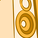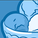# App Development

Announcements
April 22, 2PM EST: Learn about GeoOperations in Qlik Sense SaaS READ MORE
cancel
Showing results for
Did you mean:## Subtract two variables

Hi - I have the following two variables created :

vACT

IF([FINANCEACTBDGYEAR]=\$(vBudget_current_year),IF([FINANCEACTBDGMONTH]=\$(vBudget_current_month_num),NUM([ACTUALAMOUNT],'0')))

** Works Perfect

vBDGT

IF([FINANCEACTBDGYEAR]=\$(vBudget_current_year),IF([FINANCEACTBDGMONTH]=\$(vBudget_current_month_num),NUM([BUDGETAMOUNT],'0')))

** Works Perfect

I created a new variable (the variance of the two)

vACTBUDVAR

=SUM((vACT)-(vBDGT))

does not work - results in zero - any thoughts ?

1 Solution

Accepted SolutionsAuthor

HI - The below works - thanks for your guidance:

=SUM((\$(=vBDGT)))-SUM((\$(=vACT)))

2 RepliesSpecialist

I think you need to add the \$() expansion:

=SUM((\$(vACT))-(\$(vBDGT)))

You may need to add an = sign to your 2 variables or the within the \$():

=SUM((\$(=vACT))-(\$(=vBDGT)))